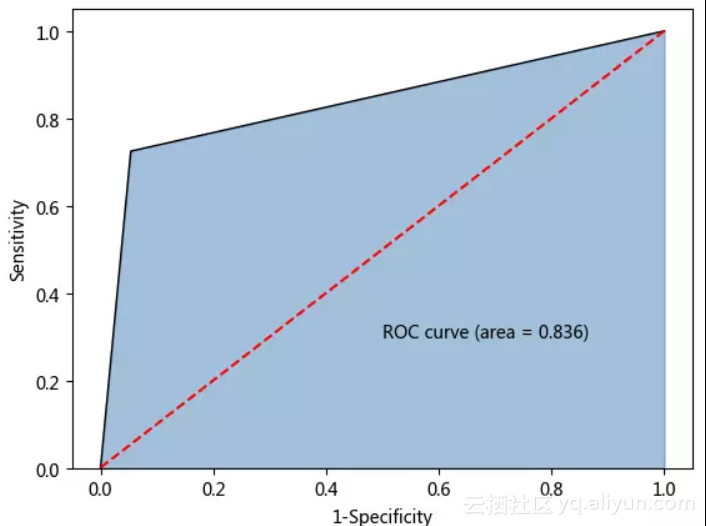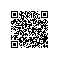# 教你用Python解决非平衡数据问题（附代码）

### SMOTE算法的介绍

SMOTE算法的基本思想就是对少数类别样本进行分析和模拟，并将人工模拟的新样本添加到数据集中，进而使原始数据中的类别不再严重失衡。该算法的模拟过程采用了KNN技术，模拟生成新样本的步骤如下：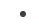采样最邻近算法，计算出每个少数类样本的K个近邻；从K个近邻中随机挑选N个样本进行随机线性插值；构造新的少数类样本；将新样本与原数据合成，产生新的训练集；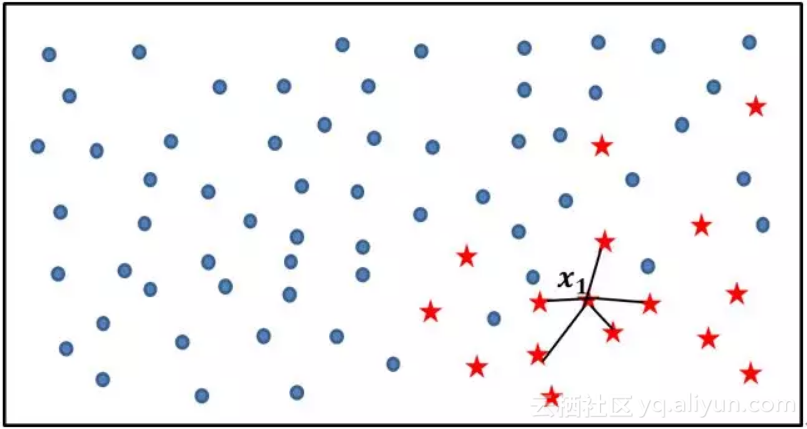利用KNN算法，选择离样本点x1最近的K个同类样本点（不妨最近邻为5）；从最近的K个同类样本点中，随机挑选M个样本点（不妨M为2），M的选择依赖于最终所希望的平衡率；对于每一个随机选中的样本点，构造新的样本点；新样本点的构造需要使用下方的公式：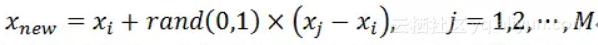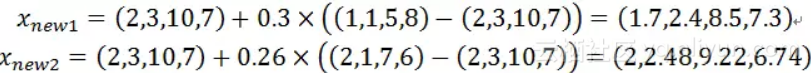重复步骤1）、2）和3），通过迭代少数类别中的每一个样本xi，最终将原始的少数类别样本量扩大为理想的比例；

SMOTE(ratio=’auto’, random_state=None, k_neighbors=5, m_neighbors=10,

out_step=0.5, kind=’regular’, svm_estimator=None, n_jobs=1)ratio：用于指定重抽样的比例，如果指定字符型的值，可以是’minority’，表示对少数类别的样本进行抽样、’majority’，表示对多数类别的样本进行抽样、’not minority’表示采用欠采样方法、’all’表示采用过采样方法，默认为’auto’，等同于’all’和’not minority’；如果指定字典型的值，其中键为各个类别标签，值为类别下的样本量；random_state：用于指定随机数生成器的种子，默认为None,表示使用默认的随机数生成器；k_neighbors：指定近邻个数，默认为5个；m_neighbors：指定从近邻样本中随机挑选的样本个数，默认为10个；kind：用于指定SMOTE算法在生成新样本时所使用的选项，默认为’regular’，表示对少数类别的样本进行随机采样，也可以是’borderline1’、’borderline2’和’svm’；svm_estimator：用于指定SVM分类器，默认为sklearn.svm.SVC，该参数的目的是利用支持向量机分类器生成支持向量，然后再生成新的少数类别的样本；n_jobs：用于指定SMOTE算法在过采样时所需的CPU数量，默认为1表示仅使用1个CPU运行算法，即不使用并行运算功能；

### 分类算法的应用实战

# 导入第三方包

import pandas as pd

import numpy as np

import matplotlib.pyplot as plt

from sklearn import model_selection

from sklearn import tree

from sklearn import metrics

from imblearn.over_sampling import SMOTE

churn.head()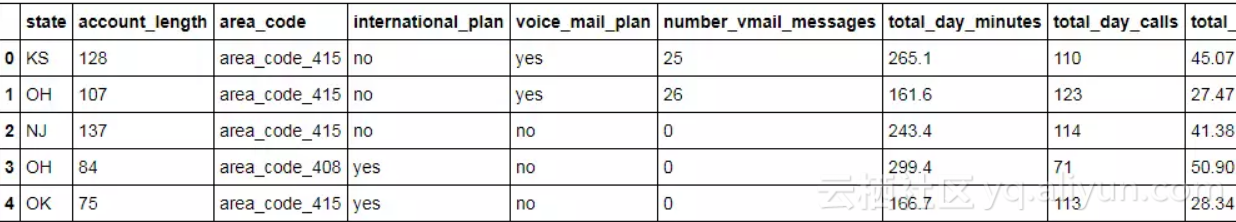# 中文乱码的处理

plt.rcParams['font.sans-serif']=['Microsoft YaHei']

# 为确保绘制的饼图为圆形，需执行如下代码

plt.axes(aspect = 'equal')

# 统计交易是否为欺诈的频数

counts = churn.churn.value_counts()

# 绘制饼图

plt.pie(x = counts, # 绘图数据

labels=pd.Series(counts.index).map({'yes':'流失','no':'未流失'}), # 添加文字标签

autopct='%.2f%%' # 设置百分比的格式，这里保留一位小数
)

# 显示图形

plt.show()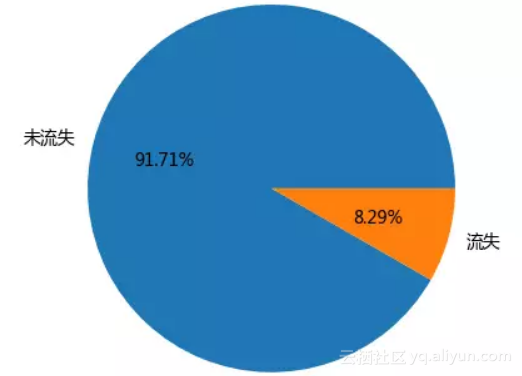# 数据清洗

# 删除state变量和area_code变量

churn.drop(labels=['state','area_code'], axis = 1, inplace = True)

# 将二元变量international_plan和voice_mail_plan转换为0-1哑变量

churn.international_plan = churn.international_plan.map({'no':0,'yes':1})
churn.voice_mail_plan = churn.voice_mail_plan.map({'no':0,'yes':1})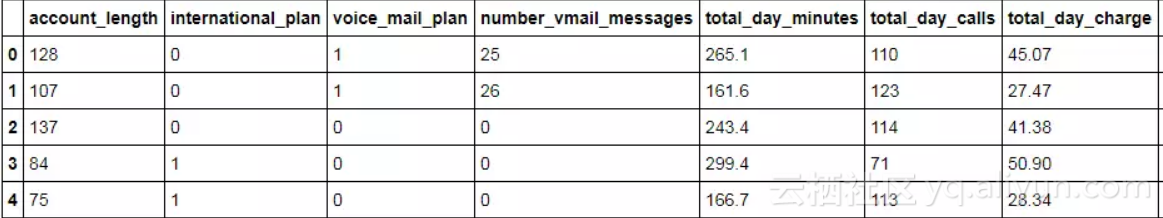# 用于建模的所有自变量

predictors = churn.columns[:-1]

# 数据拆分为训练集和测试集

X_train,X_test,y_train,y_test = model_selection.train_test_split(churn[predictors], churn.churn, random_state=12)

# 构建决策树

dt = tree.DecisionTreeClassifier(n_estimators = 300)
dt.fit(X_train,y_train)

# 模型在测试集上的预测

pred = dt.predict(X_test)

# 模型的预测准确率

print(metrics.accuracy_score(y_test, pred))

# 模型评估报告

print(metrics.classification_report(y_test, pred))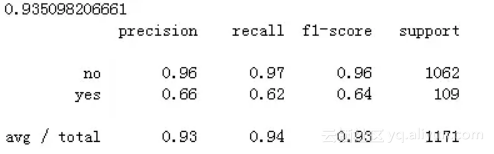# 绘制ROC曲线

# 计算流失用户的概率值，用于生成ROC曲线的数据

y_score = dt.predict_proba(X_test)[:,1]
fpr,tpr,threshold = metrics.roc_curve(y_test.map({'no':0,'yes':1}), y_score)

# 计算AUC的值

roc_auc = metrics.auc(fpr,tpr)

# 绘制面积图

plt.stackplot(fpr, tpr, color='steelblue', alpha = 0.5, edgecolor = 'black')

# 添加边际线

plt.plot(fpr, tpr, color='black', lw = 1)

# 添加对角线

plt.plot([0,1],[0,1], color = 'red', linestyle = '--')

# 添加文本信息

plt.text(0.5,0.3,'ROC curve (area = %0.3f)' % roc_auc)

# 添加x轴与y轴标签

plt.xlabel('1-Specificity')
plt.ylabel('Sensitivity')

# 显示图形

plt.show()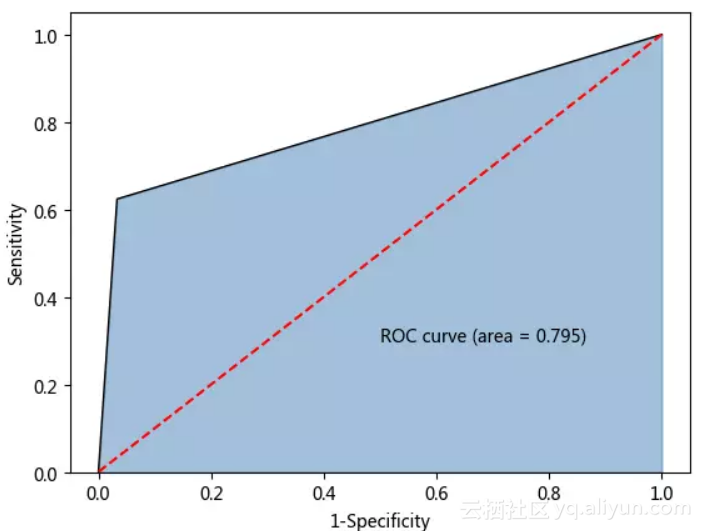# 对训练数据集作平衡处理

over_samples = SMOTE(random_state=1234)
over_samples_X,over_samples_y = over_samples.fit_sample(X_train, y_train)

# 重抽样前的类别比例

print(y_train.value_counts()/len(y_train))

# 重抽样后的类别比例

print(pd.Series(over_samples_y).value_counts()/len(over_samples_y))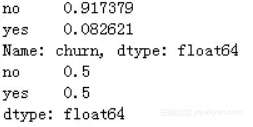# 基于平衡数据重新构建决策树模型

dt2 = ensemble.DecisionTreeClassifier(n_estimators = 300)
dt2.fit(over_samples_X,over_samples_y)

# 模型在测试集上的预测

pred2 =dt2.predict(np.array(X_test))

# 模型的预测准确率

print(metrics.accuracy_score(y_test, pred2))

# 模型评估报告

print(metrics.classification_report(y_test, pred2))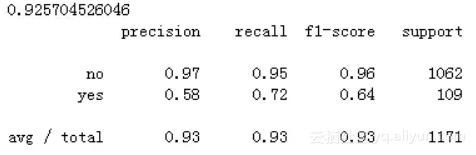# 计算流失用户的概率值，用于生成ROC曲线的数据

y_score = rf2.predict_proba(np.array(X_test))[:,1]
fpr,tpr,threshold = metrics.roc_curve(y_test.map({'no':0,'yes':1}), y_score)

# 计算AUC的值

roc_auc = metrics.auc(fpr,tpr)

# 绘制面积图

plt.stackplot(fpr, tpr, color='steelblue', alpha = 0.5, edgecolor = 'black')

# 添加边际线

plt.plot(fpr, tpr, color='black', lw = 1)

# 添加对角线

plt.plot([0,1],[0,1], color = 'red', linestyle = '--')

# 添加文本信息

plt.text(0.5,0.3,'ROC curve (area = %0.3f)' % roc_auc)

# 添加x轴与y轴标签

plt.xlabel('1-Specificity')
plt.ylabel('Sensitivity')

# 显示图形

plt.show()